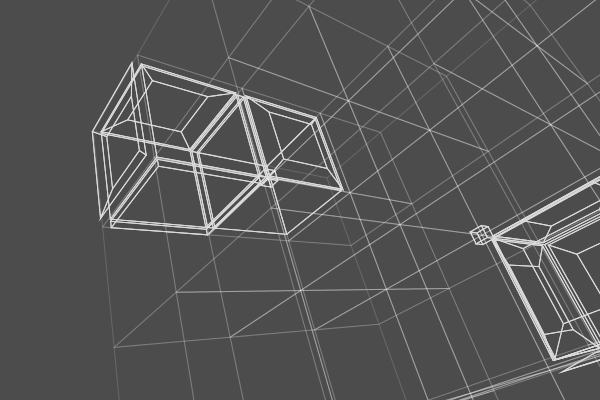DGtal  1.3.beta
io/boards/dgtalBoard3DTo2D-KSCell.cpp

Khalimsky Signed Cell Visualization (with Board3DTo2D)Khalimsky Signed Cell Visualization (with Board3DTo2D)
//#include <iostream>
#include "DGtal/io/boards/Board3DTo2D.h"
#include "DGtal/base/Common.h"
#include "DGtal/helpers/StdDefs.h"
#include "DGtal/shapes/Shapes.h"
using namespace std;
using namespace DGtal;
using namespace Z3i;
// Standard services - public :
int main()
{
Point plow(0,0,0);
Point pup(3,3,2);
Domain domain( plow, pup );
K.init( plow, pup, true );
Board3DTo2D<> board(K);
board << SetMode3D( domain.className(), "Paving" );
board << domain;
// Drawing cell of dimension 3
Cell voxelA = K.uCell(Point(1,1,1));
SCell voxelB = K.sCell(Point(1,1,3));
board << voxelB << voxelA;
// drawing cells of dimension 2
SCell surfelA = K.sCell( Point( 2, 1, 3 ) );
SCell surfelB = K.sCell( Point( 1, 0, 1 ), false );
Cell surfelC = K.uCell( Point( 1, 2, 1 ) );
SCell surfelD = K.sCell( Point( 1, 1, 0 ) );
Cell surfelE = K.uCell( Point( 1, 1, 2 ) );
board << surfelA << surfelB << surfelC << surfelD << surfelE;
Cell linelA = K.uCell(Point(2,1 ,2));
SCell linelB = K.sCell(Point(2,2 ,1));
SCell linelC = K.sCell(Point(1,2 ,2), false);
board << linelA << linelB << linelC;
Cell center = K.uCell(Point(5,5,5));
// Testing display of oriented surfels:
SCell ssurfelXZ = K.sCell( Point( 5, 6, 5 ), false );
SCell ssurfelXY = K.sCell( Point( 5, 5, 6 ), false );
SCell ssurfelZY = K.sCell( Point( 6, 5, 5 ), false );
board << center;
SCell ssurfelXZo = K.sCell( Point( 5, 4, 5 ), false );
SCell ssurfelXYo = K.sCell( Point( 5, 5, 4 ), false );
SCell ssurfelZYo = K.sCell( Point( 4, 5, 5 ), false );
board << ssurfelXZ << ssurfelXY << ssurfelZY;
board << ssurfelXZo << ssurfelXYo << ssurfelZYo;
// Testing display oriented pointels
Cell pointelA = K.uCell(Point(2, 2, 2));
SCell pointelB = K.sCell(Point(4, 4, 4), true);
SCell pointelC = K.sCell(Point(6, 4, 4), false);
SCell linelAC = K.sCell(Point(5, 4, 4), false);
board << pointelA << pointelB << pointelC << linelAC;
/*board << CameraPosition(2.69044, 1.73705, -1.89961)
<< CameraUpVector(0.48806, -0.869135, 0.0800053);*/
board << CameraPosition(3.49239, 3.04746, -1.40276)
<< CameraUpVector(0.516135, -0.84913, -0.112173);
//board << SetMode3D(board.className(), "WireFrameMode");
board.saveCairo("dgtalBoard3DTo2D-KSCell.png", Board3DTo2D<Space, KSpace>::CairoPNG, 600, 400);
}
// //
DGtal::KhalimskySpaceND::uCell
Cell uCell(const PreCell &c) const
From an unsigned cell, returns an unsigned cell lying into this Khalismky space.
DGtal::HyperRectDomain< Space >
DGtal::detail::center
Point center(const std::vector< Point > &points)
Definition: QuickHullKernels.h:66
DGtal::KhalimskySpaceND::init
bool init(const Point &lower, const Point &upper, bool isClosed)
Specifies the upper and lower bounds for the maximal cells in this space.
DGtal::KhalimskySpaceND::sCell
SCell sCell(const SPreCell &c) const
From a signed cell, returns a signed cell lying into this Khalismky space.
DGtal::HyperRectDomain::className
std::string className() const
K
KSpace K
Definition: testCubicalComplex.cpp:62
DGtal::SignedKhalimskyCell
Represents a signed cell in a cellular grid space by its Khalimsky coordinates and a boolean value.
Definition: KhalimskySpaceND.h:208
DGtal::Board3DTo2D
Class for PDF, PNG, PS, EPS, SVG export drawings with Cairo with 3D->2D projection.
Definition: Common.h:162
DGtal
DGtal is the top-level namespace which contains all DGtal functions and types.
CameraDirection class to set camera direction.
Definition: DrawWithBoard3DTo2DModifier.h:90
DGtal::SetMode3D
Modifier class in a Display3D stream. Useful to choose your own mode for a given class....
Definition: DrawWithDisplay3DModifier.h:73
main
int main(int argc, char **argv)
Definition: testArithmeticDSS-benchmark.cpp:147
DGtal::CameraPosition
CameraPosition class to set camera position.
Definition: DrawWithBoard3DTo2DModifier.h:70
domain
Domain domain
Definition: testProjection.cpp:88
DGtal::CameraUpVector
CameraUpVector class to set camera up-vector.
Definition: DrawWithBoard3DTo2DModifier.h:110
DGtal::Board3DTo2D::saveCairo
void saveCairo(const char *filename, CairoType type, int bWidth, int bHeight)
Point
MyPointD Point
Definition: testClone2.cpp:383
DGtal::KhalimskyCell< dim, Integer >
DGtal::KhalimskySpaceND
Aim: This class is a model of CCellularGridSpaceND. It represents the cubical grid as a cell complex,...
Definition: KhalimskySpaceND.h:64